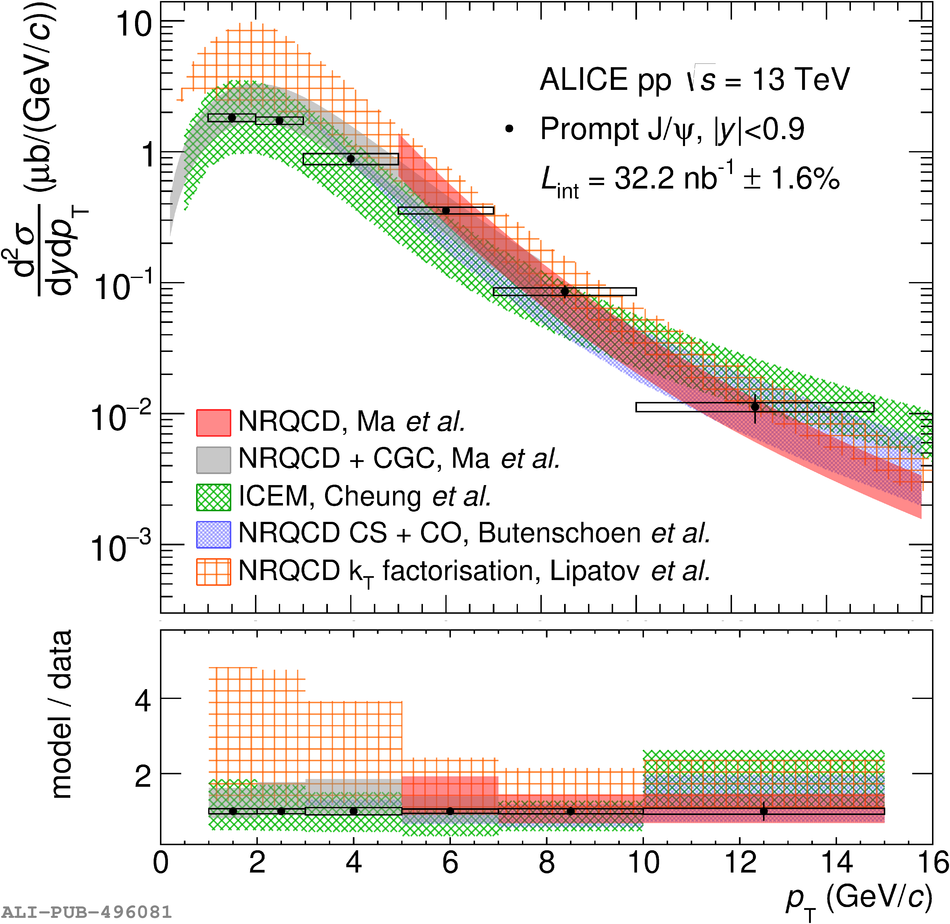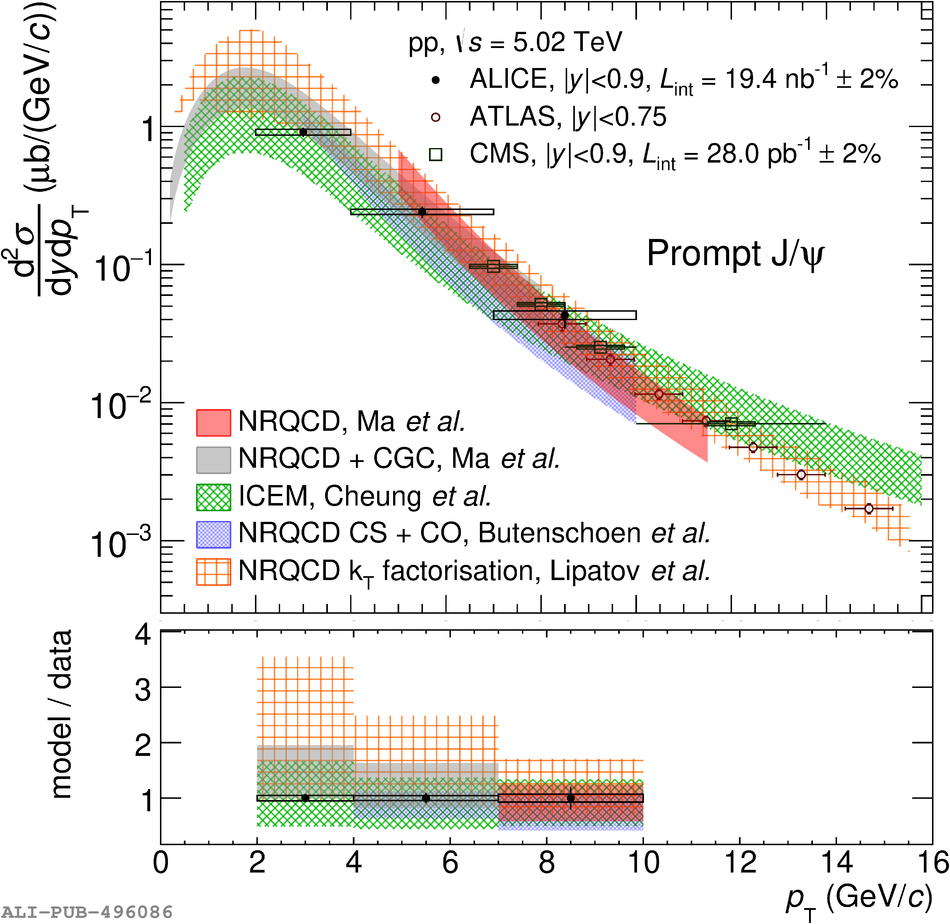# Figure 4

 The $\frac{{\rm d}^2\sigma}{{\rm d}y {\rm d}\pt}$ of prompt \pJPsi measured by the ALICE collaboration in pp collisions at \s = 13 TeV (left panel) and \s = 5.02 TeV (right panel). The error bars (boxes) represent the statistical (systematic) uncertainty. The results at \s = 5.02 TeV are compared with similar measurements from CMS  and ATLAS  at high \pt. Uncertainties due to the luminosity are not included in the boxes, except in the case of ATLAS. The results are compared with calculations from NLO NRQCD , NRQCD+CGC , and from ICEM . Bottom panels show the ratios of the models to ALICE results. The uncertainty bands represent the relative uncertainty from each model whereas the points centered around unity refer to relative statistical and systematic uncertainties on ALICE data points.